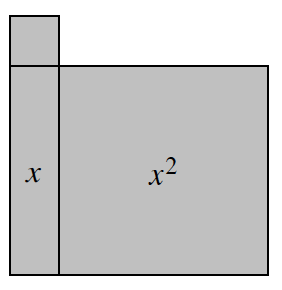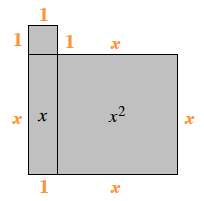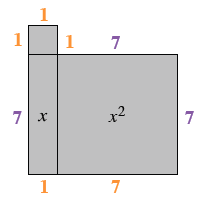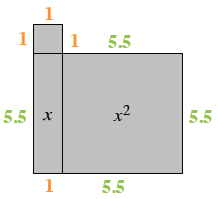### Home > CC2MN > Chapter 6 Unit 6B > Lesson CC2: 6.1.4 > Problem6-45

6-45.On your paper, sketch the algebra tile shape at right. Write an expression for the perimeter, and then find the perimeter for each of the given values of $x$. Homework Help ✎

The perimeter is the sum of all the sides. Mark each side with its length to help you find the perimeter.

Combine like terms to form an expression of the perimeter.1. $x=7$ cm

Substitute $7$ for $x$ in the algebra tile shape or the expression you found to find the perimeter for this given value.

1. $x=5.5$ cm

Substitute $5.5$ for $x$ in the algebra tile shape or the expression you found to find the perimeter for this given value.